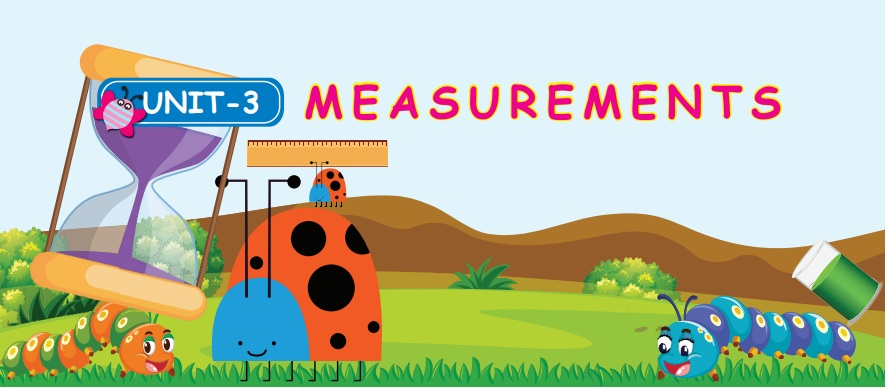Home | | Maths 4th Std | Recall

# Recall

A one dimension quantity − length. Two dimension quantity − length and breadth. When we expand 1 cm from the surface of 2D, it forms 3D. Three dimensional quantity is called as volume.

UNIT−3

MEASUREMENTSRecall:

VolumeA one dimension quantity − length and it is represented asTwo dimension quantity − length and breadth. Which can be represented asThe space ocupied here: 1cm x 1cm = 1 cm2.

Two dimensional quantity is called as Area.

Definition of volume

When we expand 1 cm from the surface of 2D, it forms 3D.The space occupied here: 1cm x 1cm x 1cm = 1 cm3.

Three dimensional quantity is called as volume.

So, volume is the space occupied by any 3D object (cone, cube, cuboid and cylinder).

Tags : Measurements | Term 3 Chapter 3 | 4th Maths , 4th Maths : Term 3 Unit 3 : Measurements
Study Material, Lecturing Notes, Assignment, Reference, Wiki description explanation, brief detail
4th Maths : Term 3 Unit 3 : Measurements : Recall | Measurements | Term 3 Chapter 3 | 4th Maths